Note: This is an archvied version of our old webpage. Some links might be broken. The current one can be found here.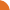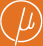Chair for Foundations of Software Reliability and Theoretical Computer Science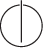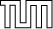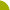Publications - Solving Systems of Positive Polynomial EquationsReference: Stefan Kiefer. Solving systems of positive polynomial equations. PhD thesis, Technische Universität München, 2009. Abstract: We consider equation systems of the form X_1 = f_1(X_1, ..., X_n), ..., X_n = f_n(X_1, ..., X_n) where f_i(X_1, ..., X_n) is, for all i in 1, ..., n, an expression built up from real-valued variables X_1, ..., X_n, nonnegative real constants, and the operators multiplication, addition, minimum and maximum. We call such an equation system positive and denote it in vector form by X = f(X). The least solution is called mu, i.e., mu is the least fixed point of f. Positive equation systems appear naturally in the analysis of stochastic models like stochastic context-free grammars (with numerous applications to natural language processing and computational biology), probabilistic programs with procedures, web-surfing models with back buttons, branching processes, and termination games. The solution mu of a positive equation system X = f(X) is of central interest for these models. Efficient methods to compute mu are the main subject of this thesis. For positive equation systems without minimum or maximum operator, Newton's method for approximating a zero of a differentiable function can be applied to approximate mu. In the first part of the thesis, we study in detail the convergence speed of Newton's method for such equation systems and show, in particular, that Newton's method converges at least linearly to mu. We also give concrete bounds on the convergence rate. To compute the least fixed point of general positive equation systems with minimum and maximum operators, Newton's method cannot be directly used. In the second part, we suggest two algorithms that combine Newton's method with linear programming. We show that these methods converge linearly to mu and give bounds on the convergence rate. We also show that one of those methods can be used to compute near-optimal strategies for the game associated with positive equation systems. Suggested BibTeX entry: @phdthesis{Kie09,     author = {Stefan Kiefer},     school = {Technische Universit\"{a}t M\"{u}nchen},     title = {Solving Systems of Positive Polynomial Equations},     year = {2009} }